Drop a Query

# PySpark

## PySpark Exercises – 101 PySpark Exercises for Data Analysis

101 PySpark exercises are designed to challenge your logical muscle and to help internalize data manipulation with python’s favorite package for data analysis. The questions are of 3 levels of difficulties with L1 being the easiest to L3 being the hardest. You might also like to try out: 101 Pandas Exercises for Data Analysis 101 …

## PySpark OneHot Encoding – Mastering OneHot Encoding in PySpark and Unleash the Power of Categorical Data in Machine Learning

Let’s dive deep into OneHot Encoding in PySpark, exploring its benefits in machine learning and walking you through practical example with code. As machine learning continues to gain traction in the world of data science, the need for efficient data preprocessing has never been more crucial. One such preprocessing technique is OneHot Encoding, which allows …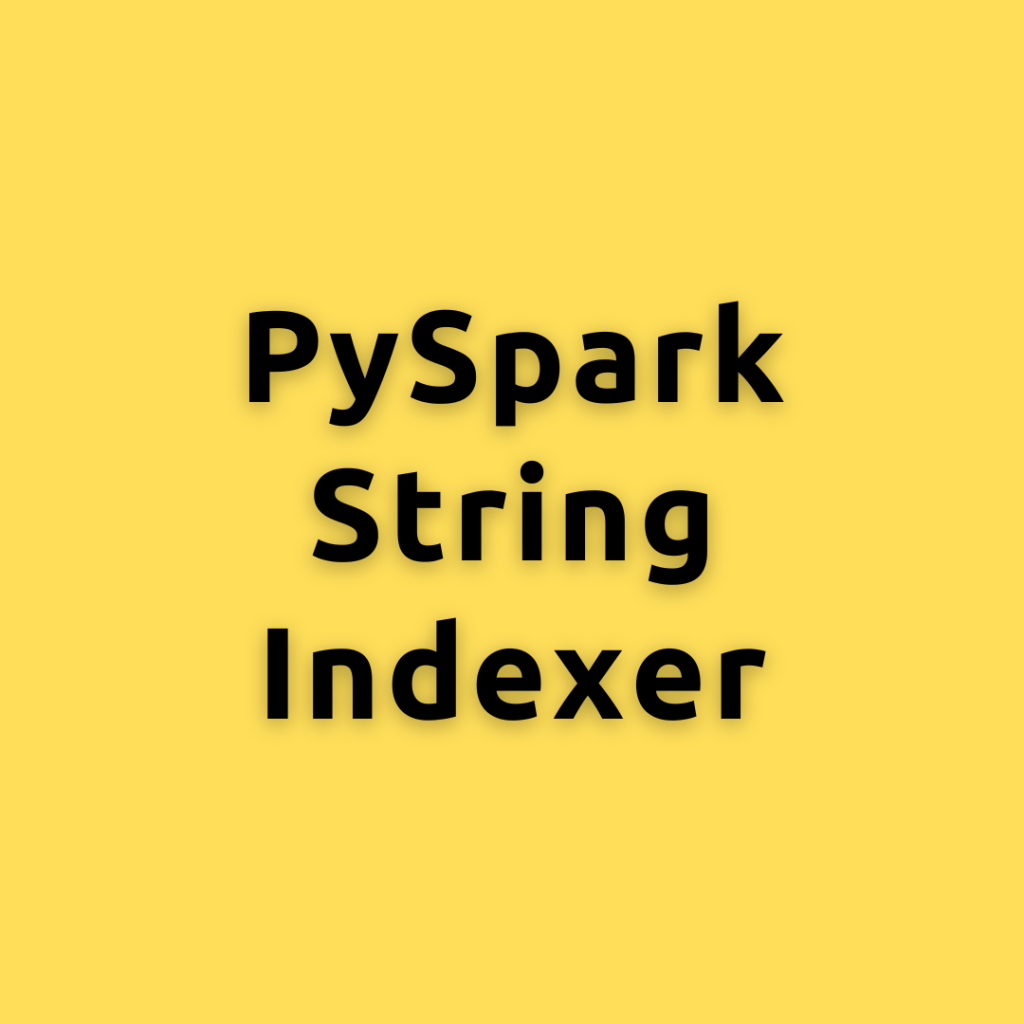## PySpark StringIndexer – A Comprehensive Guide to master PySpark StringIndexer

Deep understanding of PySpark’s StringIndexe, how it works, and how to effectively use it in your PySpark workflow Machine learning practitioners often encounter categorical data that needs to be transformed into a numerical format. We will delve into PySpark’s StringIndexer, an essential feature that converts categorical string columns into numerical indices. This guide will provide …## PySpark Outlier Detection and Treatment – A Comprehensive Guide How to handle Outlier in PySpark

Let’s dive deep into how to identify and treat outliers in PySpark, a popular open-source, distributed computing system that provides a fast and general-purpose cluster-computing framework for big data processing. Outliers are unusual data points that do not follow the general trend of a dataset. They can heavily influence the results of data analysis, predictive …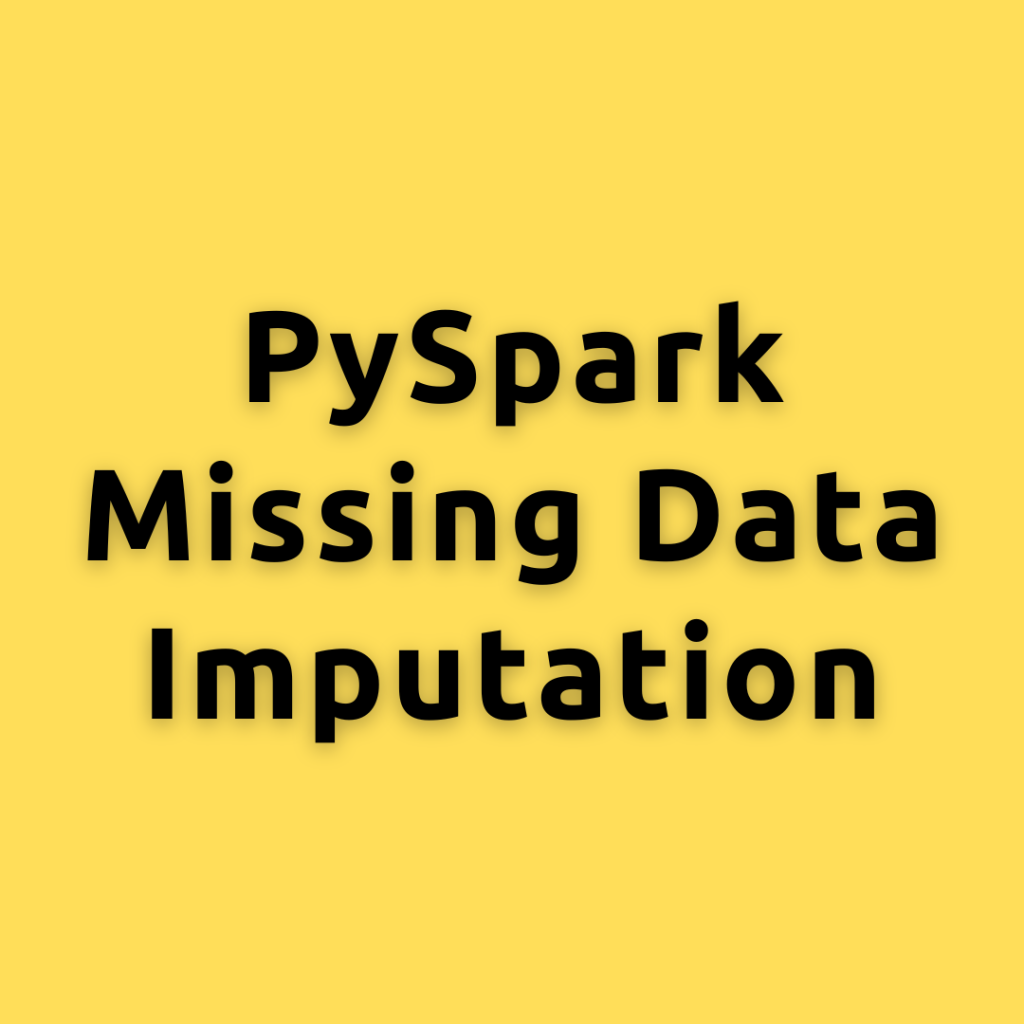## PySpark Missing Data Imputation – How to handle missing values in PySpark

Handling missing data is an essential step in the data preprocessing pipeline. let’s explore various methods to impute missing values in PySpark, a popular distributed data processing framework. We will discuss different techniques, such as mean, median, mode imputation, and using machine learning algorithms to fill in missing values. By the end of this post, …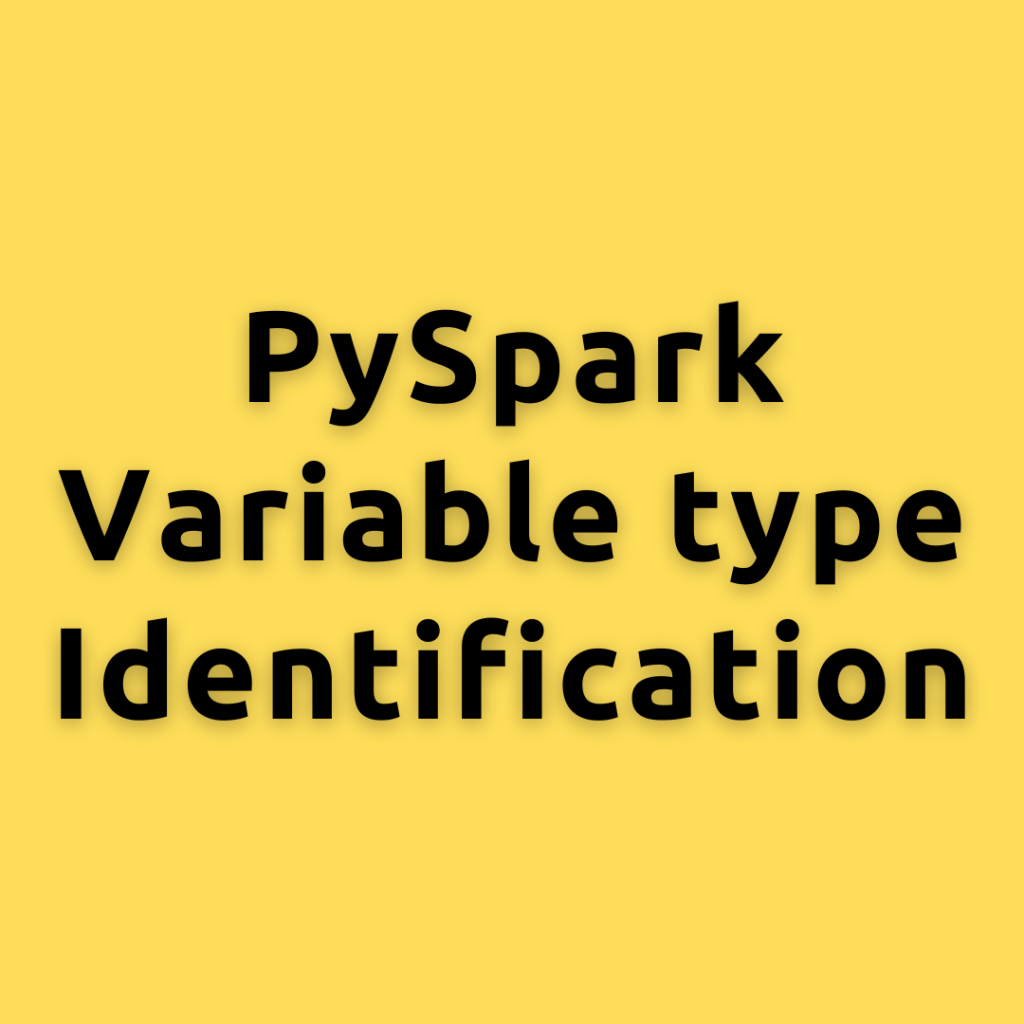## PySpark Variable type Identification – A Comprehensive Guide to Identifying Discrete, Categorical, and Continuous Variables in Data

Let’s Explore what are discrete, categorical, and continuous variables, their identification techniques, and their importance in machine learning and statistical modeling. Data preprocessing is a critical step in machine learning and statistical modeling. Before diving into model building, it is essential to understand and identify the types of variables present in the dataset. Furthermore, I …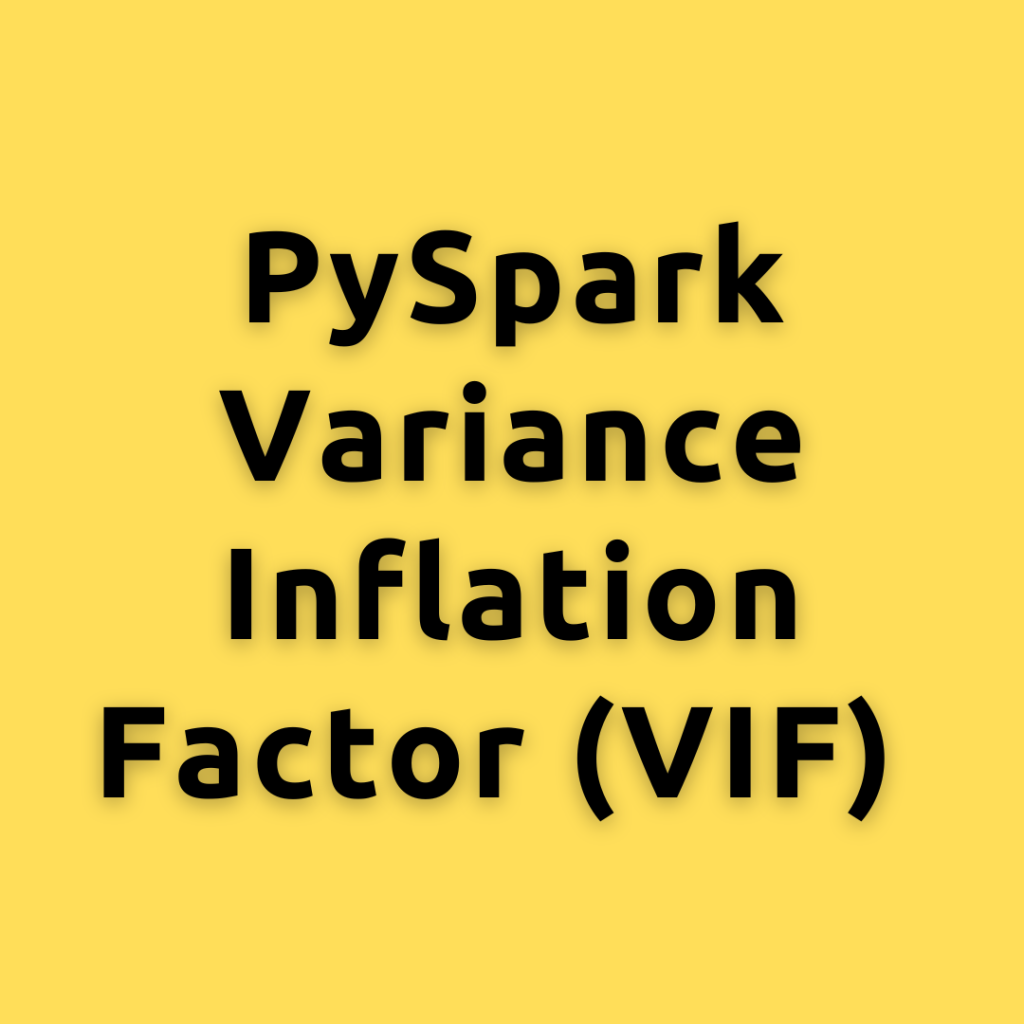## PySpark Variance Inflation Factor (VIF) – Understanding of VIF and how it can help you improve your regression models.

VIF concept is critical for understanding multicollinearity in regression models, let’s break down the concept into simple terms, explain how to calculate VIF, and discuss its practical uses What is Variance Inflation Factor (VIF)? VIF is a measure that helps us understand the extent of multicollinearity in a multiple regression model. Multicollinearity occurs when two …## PySpark Chi-Square Test – Understanding Chi-Square Test a Deep Dive with PySpark

Let’s explore the uses of Chi-Square in statistics and machine learning, and then demonstrate how to calculate the Chi-Square statistic in PySpark in different ways. Let’s dive into the world of statistics and machine learning, focusing on the Chi-Square Test. This statistical test is an essential tool for many data-driven applications and is widely used …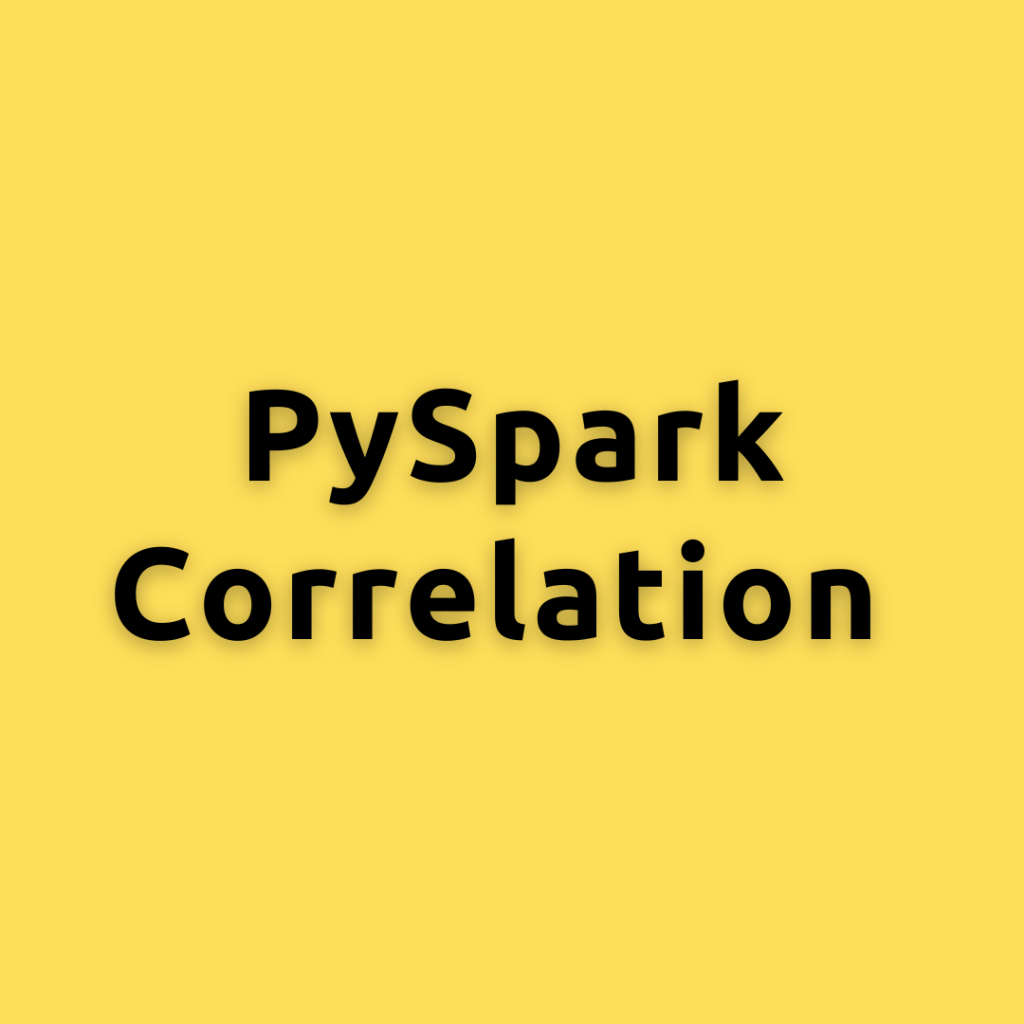## PySpark Correlation – Understanding Correlation a Deep Dive with PySpark

Lets dive into the concept of correlation, explore how to calculate it using PySpark in different ways, and discuss its applications in statistics and machine learning. In the data-driven world we live in, correlation is a key concept that is frequently used in various fields, including statistics and machine learning. Understanding the relationship between variables …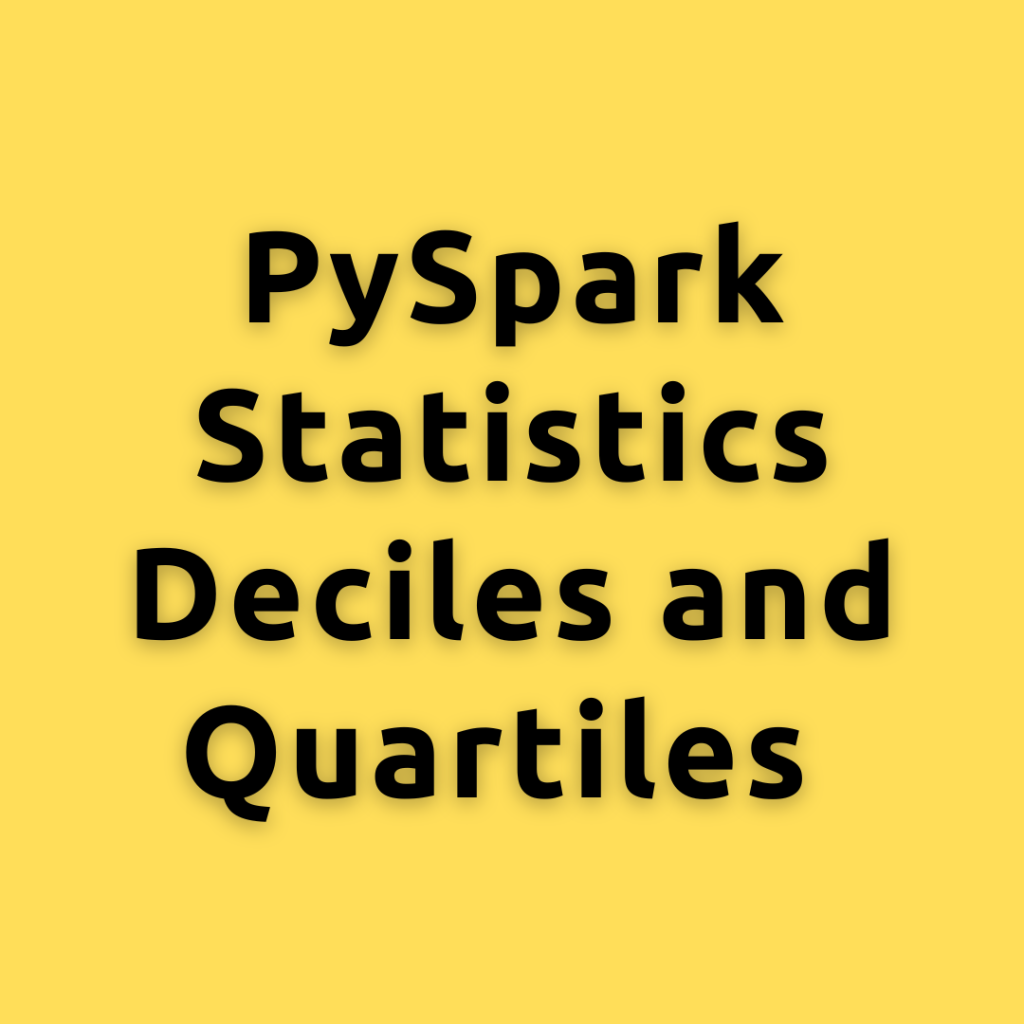## PySpark Statistics Deciles and Quartiles – Understanding Deciles and Quartiles a Deep Dive with PySpark

Let’s dive into the concept of deciles and quartiles and how to calculate them in PySpark. When analyzing data, it’s important to understand the distribution of the data. One way to do this is by calculating the deciles and quartiles. What are Deciles? Deciles divide a set of data into 10 equal parts. For example, …## PySpark Statistics Variance – Understanding Variance a Deep Dive with PySpark

Let’s dive into the concept Variance, the formula to calculate Variance, and how to compute in PySpark, a powerful open-source data processing engine. When analyzing data, it’s essential to understand the underlying concepts of variability and dispersion. Two key measures for this are variance What is Variance? Variance is a measure of dispersion in a …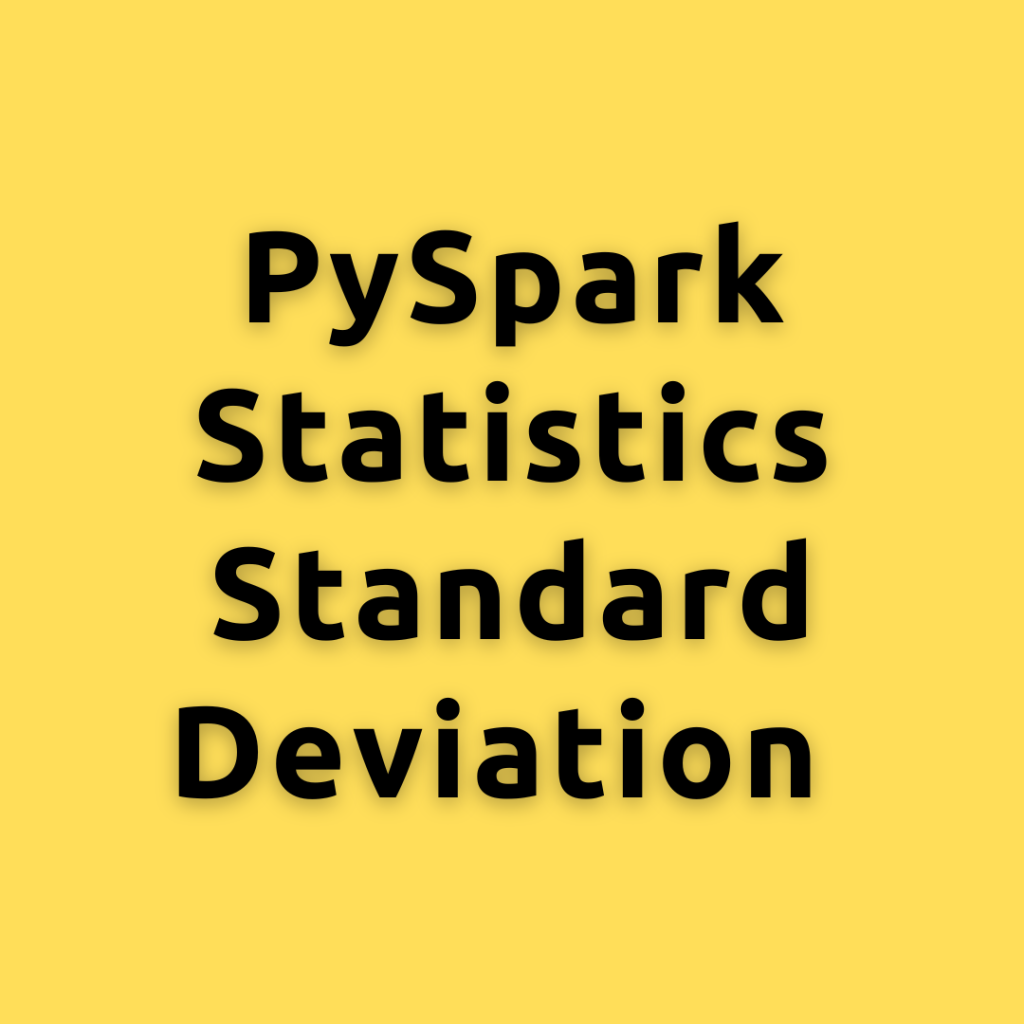## PySpark Statistics Standard Deviation – Calculating the Standard Deviation in PySpark a Comprehensive Guide for Everyone

Lets dive into the concept of Standard Deviation, its importance in statistics and machine learning, and explore different ways to calculate it using PySpark How to Calcualte Standard Deviation? Standard Deviation is a measure that quantifies the amount of variation or dispersion in a set of data values. It helps in understanding how far individual …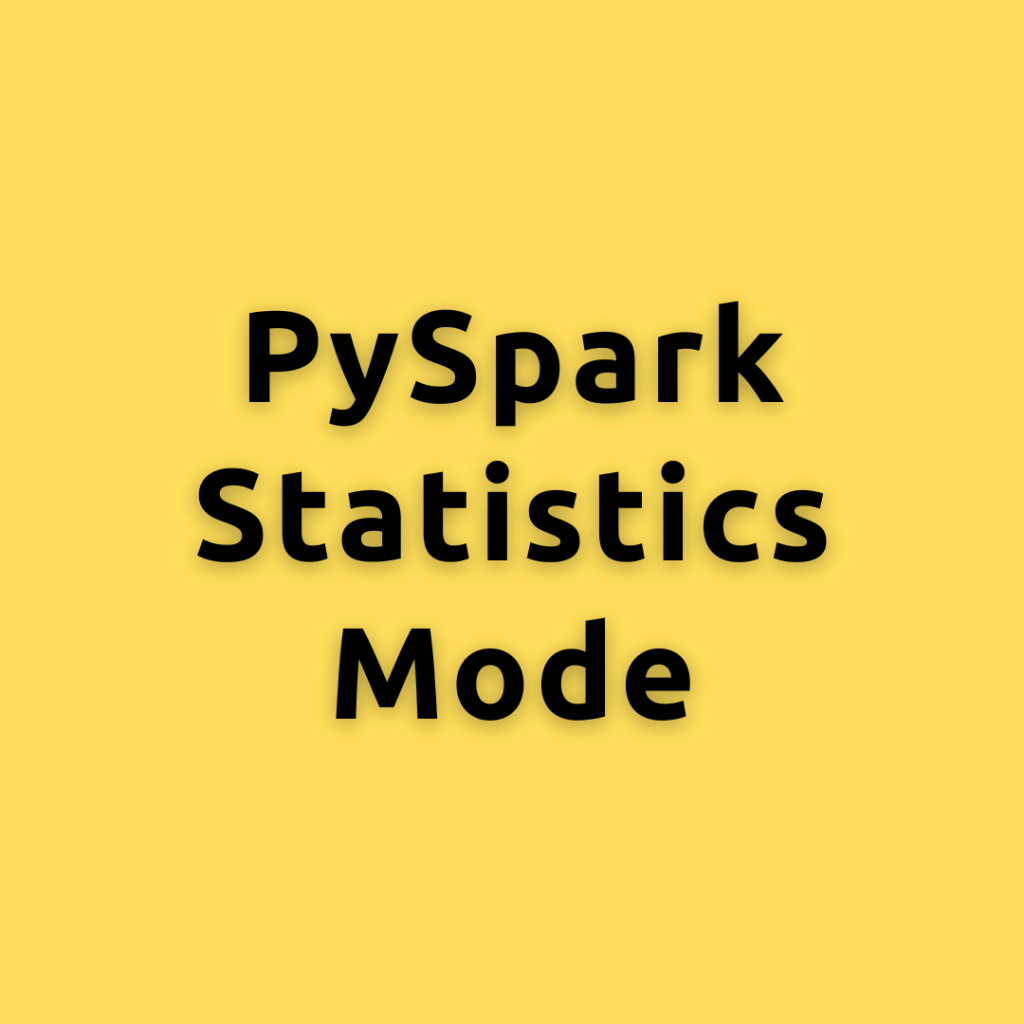## PySpark Statistics Mode – Calculating the Mode in PySpark a Comprehensive Guide for Everyone

Lets explore different ways of calculating the Mode using PySpark, helping you become an expert Mode is the value that appears most frequently in a dataset. It is a measure of central tendency, similar to mean and median, but focuses on the most common value(s) in the data. Mode can be applied to both numerical …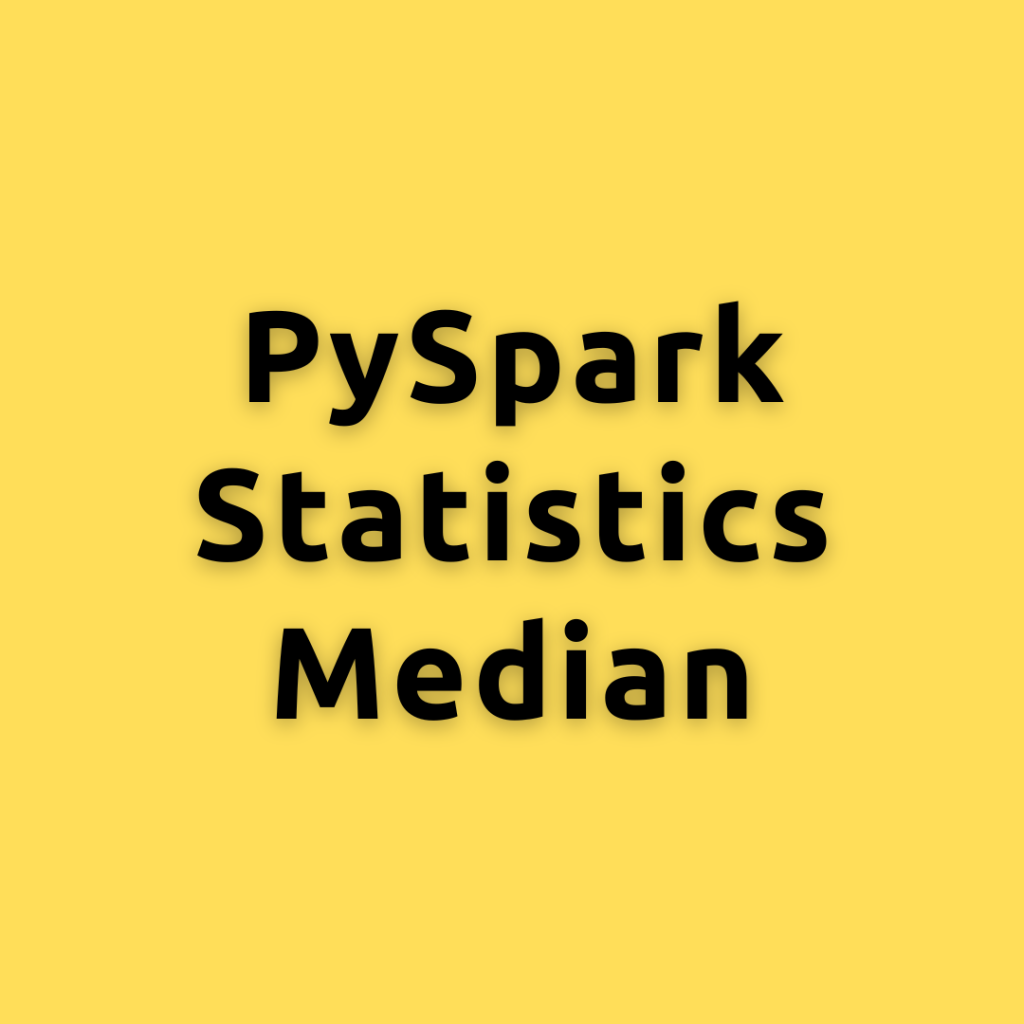## PySpark Statistics Median – Calculating the Median in PySpark a Comprehensive Guide for Everyone

Lets explore different ways of calculating the Median using PySpark, helping you become an expert As data continues to grow exponentially, efficient data processing becomes critical for extracting meaningful insights. PySpark, an Apache Spark library, enables large-scale data processing in Python. How to Calcualte Median? The median is a measure of central tendency that represents …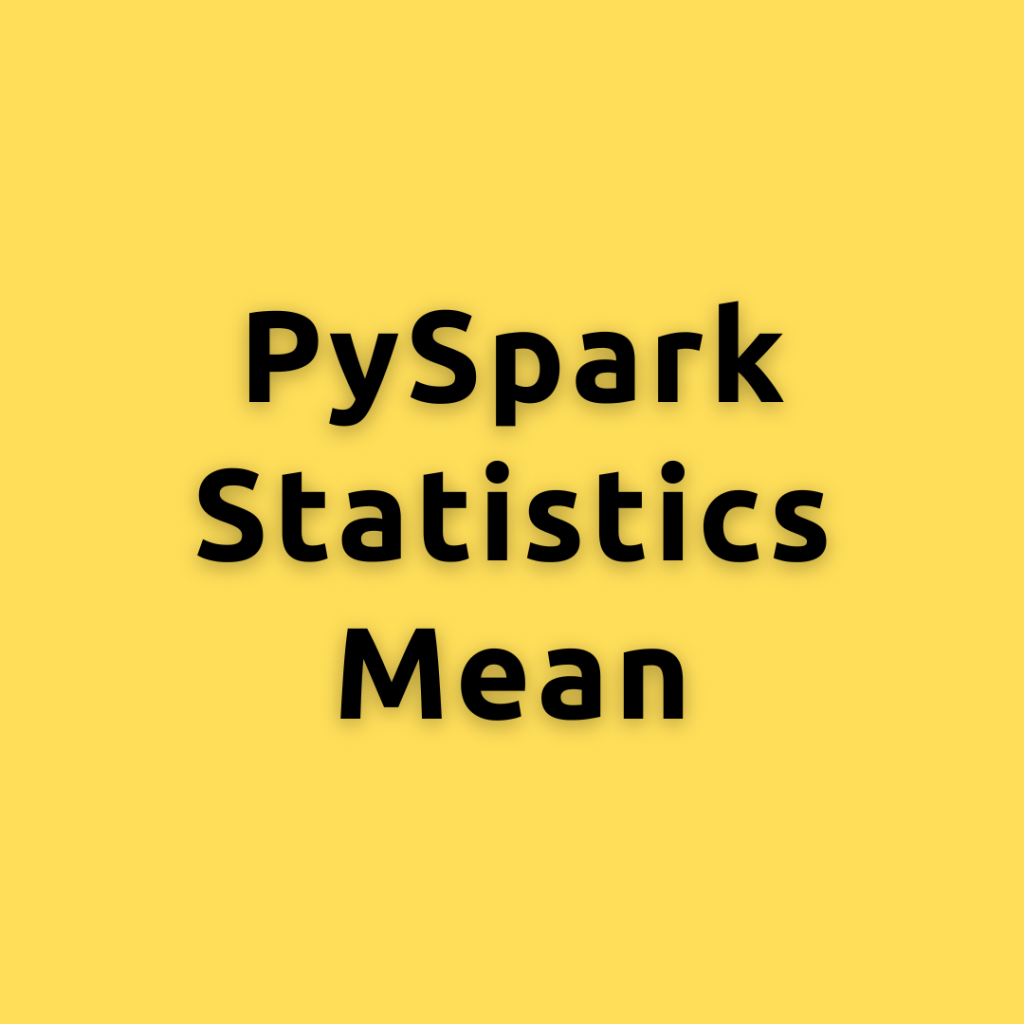## PySpark Statistics Mean – Calculating the Mean Using PySpark a Comprehensive Guide for Everyone

Lets explore different ways of calculating the mean using PySpark, helping you become an expert in no time As data continues to grow exponentially, efficient data processing becomes critical for extracting meaningful insights. PySpark, an Apache Spark library, enables large-scale data processing in Python. Concept of Mean: The mean, also known as the average, is …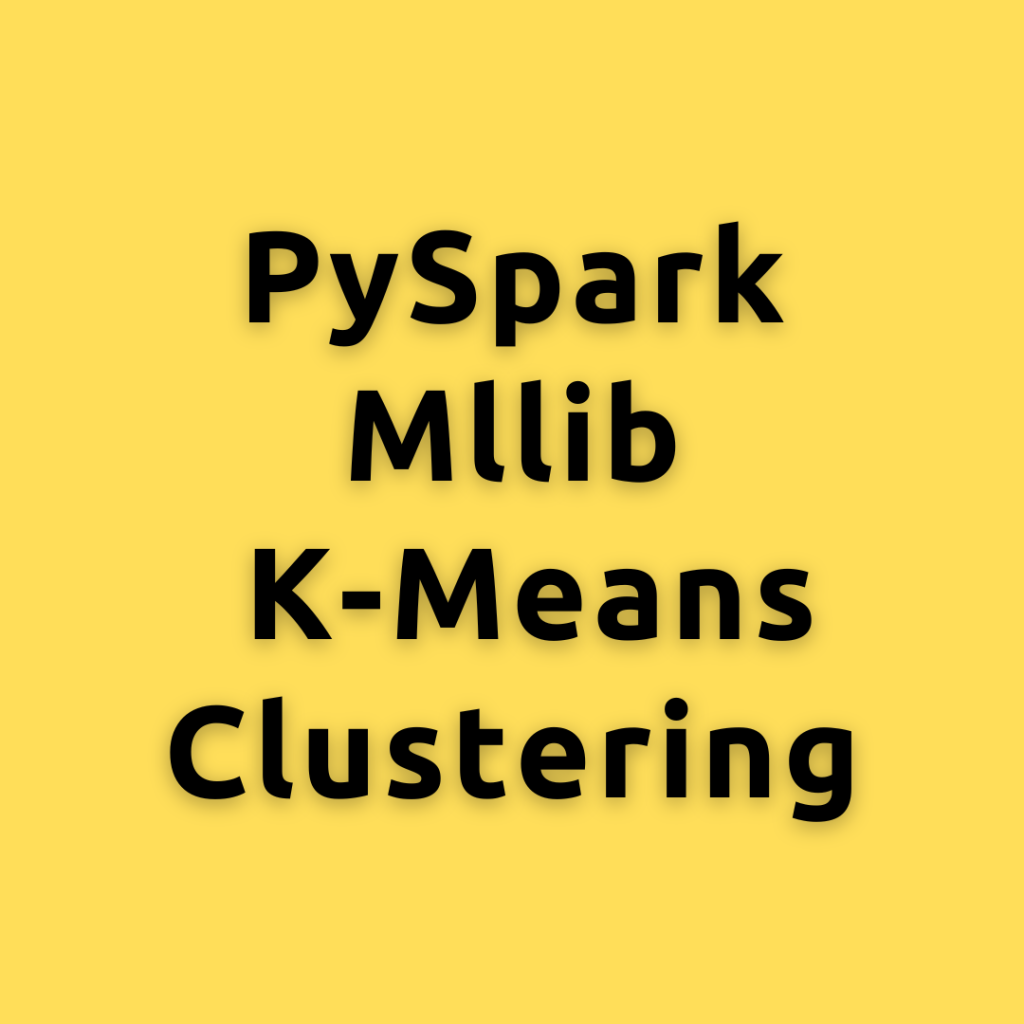## PySpark Mllib K-Means Clustering – Mastering K-means Clustering with PySpark MLlib and Example Code

Lets explore K-means clustering using PySpark’s MLlib library in-depth. PySpark is an open-source Python library that facilitates distributed data processing and offers a simple way to run machine learning algorithms on large-scale data. K-means clustering is a widely-used unsupervised machine learning algorithm that partitions a dataset into K distinct clusters based on the features of …## PySpark Gradient Boosting model – Building and Evaluating Gradient Boosting model using PySpark MLlib: A Step-By-Step Guide

Lets discuss how to build and evaluate Gradient Boosting model using PySpark MLlib and cover key aspects such as hyperparameter tuning and variable selection, providing example code to help you along the way. Gradient Boosting is a powerful machine learning technique that combines multiple weak learners to create a strong predictor. Pyspark MLlib is a …## PySpark Random Forest – Building and Evaluating Random Forest Models using PySpark MLlib: A Step-By-Step Guide

Lets discuss how to build and evaluate Random Forest models using PySpark MLlib and cover key aspects such as hyperparameter tuning and variable selection, providing example code to help you along the way. Random Forest is an ensemble machine learning algorithm that can be used for both classification and regression tasks. PySpark is the Python …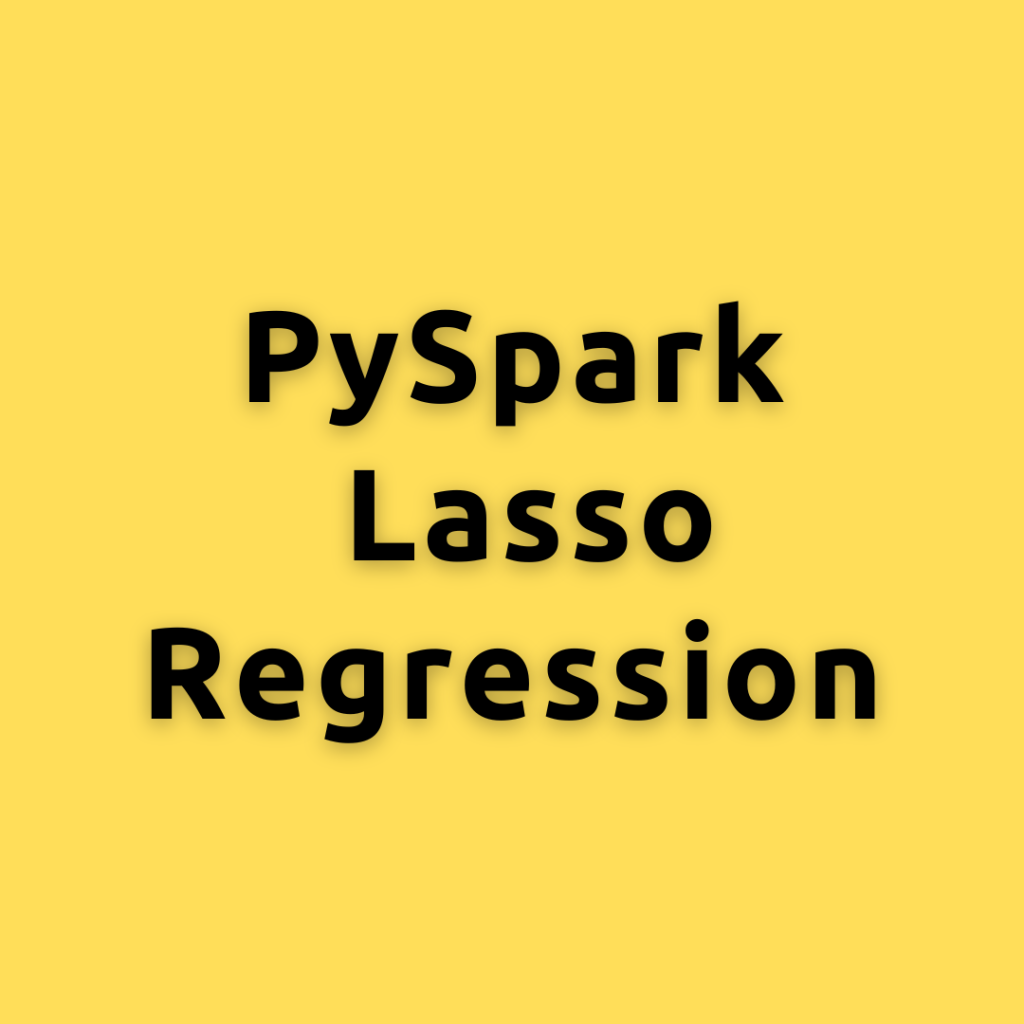## PySpark Lasso Regression – Building, Tuning, and Evaluating Lasso Regression with PySpark MLlib

Lets explore how to build, tune, and evaluate a Lasso Regression model using PySpark MLlib, a powerful library for machine learning and data processing in Apache Spark. Lasso regression is a popular machine learning algorithm that helps to identify the most important features in a dataset, allowing for more effective model building. In this blog …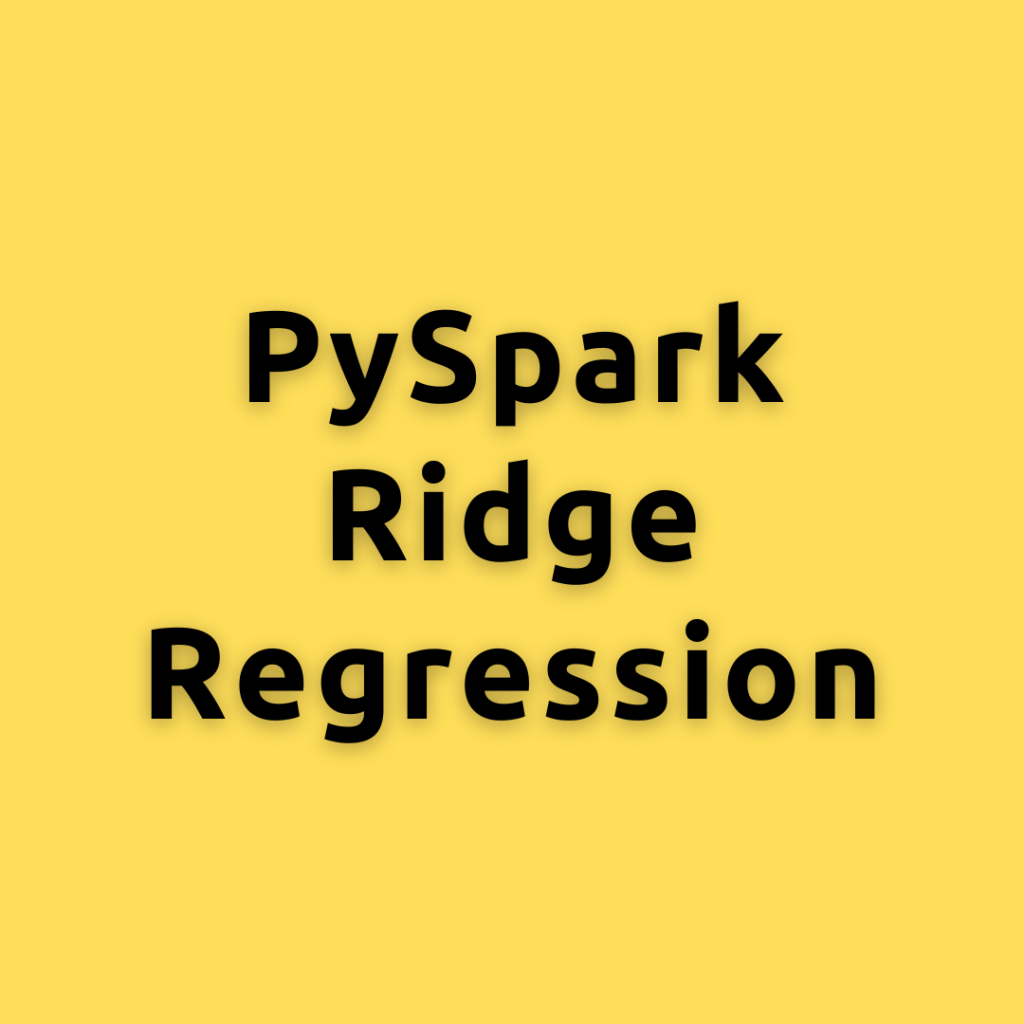## PySpark Ridge Regression – Building, Tuning, and Evaluating Ridge Regression with PySpark MLlib

Lets explore how to build, tune, and evaluate a Ridge Regression model using PySpark MLlib, a powerful library for machine learning and data processing in Apache Spark. Ridge Regression is an extension of linear regression that includes a regularization term to minimize the magnitude of the model’s coefficients and prevent overfitting. We will cover the …Course Preview

## Machine Learning A-Z™: Hands-On Python & R In Data Science

### Free Sample Videos:#### Machine Learning A-Z™: Hands-On Python & R In Data Science#### Machine Learning A-Z™: Hands-On Python & R In Data Science#### Machine Learning A-Z™: Hands-On Python & R In Data Science#### Machine Learning A-Z™: Hands-On Python & R In Data Science